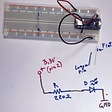# High-performance mathematical paradigms in Python

For any data-intensive discipline, one of the major overheads is making the scientific and numerical computations faster. With performance-critical application and data processing pipeline, comes the need for optimal paradigm implementation and choosing the right set of libraries. After working on science(metabolomics) and finance projects, I decided to compile these tips and tricks which I developed and learned.

The motivation behind this entire post is a line which my Mentor at Elucidata asked me to assimilate and put into practice:

There is a difference between writing code in python and writing pythonic code.

This post compiles best(debatable) practices around some of the most used data science operations. For example, evaluating complex mathematical expressions, initializing ndarrays using numpy vectorization implementation, parallel calculation, and multithreading.

## Functions defining mathematical expressions

Dealing with a large array of numbers is always a challenging task and if there is a numerical operation to be run over those elements, the story changes altogether.

Let’s say there is a mathematical expression to be evaluated over an array with 100,000 numbers:
y = ⎷|sin(x) + cos(x)|

Creating a list of numbers using the range function,

`n = 1000000data_num = range(n)`

Let’s look at the variety of implementations and we’ll compare all these at the end using the timeit library:

1. A very simple and straight-forward approach is to loop over the entire dataset and appends the output from the function f(x)(defined above)to the output list object.
`otpt = []for x in data_list:     otpt.append(f(x))`

2. We can make use of the iterators :

`return [f(x) for x in data_list]`

3. The same can be achieved using the python’s eval() method:

`exp = 'abs(cos(x) + sin(x)) ** 0.5'  return [eval(exp) for x in data_list]`

4. We can implement the same algorithm using the numpy vectorization tack.

`import numpy as npdata_np = np.arange(1000000) def f4(data_np):      """using the numpy vectorization     """    return (np.abs(np.cos(data_np) + sin(data_np)) ** 0.5)`

5. Another library which is specialized to evaluate such numerical expressions is numexpr. The reason, why this library turns out to be very handy is because of the built-in multithreading support.

`import numexpr as neex = 'abs(cos(a) + sin(a)) ** 0.5'ne.set_num_threads(5) return ne.evaluate(ex)`

We have listed 5 different paradigms to perform the same operation. It’s time to check if they have resulted in the same output or not.

Recording the output of each function as otpt1, otpt2 so on and so forth.

We can use the numpy’s allclose() method to check the two ndarray objects.

`np.allclose(otpt1, otpt2)np.allclose(otpt1, otpt3)np.allclose(otpt1, otpt4)np.allclose(otpt1, otpt5)`

They should all return true for us to look at the most important question which is the focal point of this blog:

How each of these different paradigms compares with respect to processing time. Here is the function which will help us get the timings of each function.

After passing the required arguments to the above function which uses the timeit repeat to evaluate the time and sort the values observed.

`function_list = ['f1', 'f2', 'f3', 'f4', 'f5']data_com_list =  ['data_num', 'data_num', 'data_num', 'data_np', 'data_np']record_comp_time(function_list, data_com_list)`

We get the following performance speeds.

As can be clearly seen from the comparison results, function using numexpr produces the output in record time followed by the function 4 which is the function using numpy library.

Here is the link to my GitHub repo for the entire code base:

## Conclusion

For every data intensive operation, python has a number of ways to improve the performance of your code. Given a problem, optimality can be worked upon by using the right combination of different paradigms and choice of libraries. Besides paradigms, there are some very useful libraries which improves the execution speed of the python code.

Just like, numexpr has a great range of operations which makes numerical evaluation smoother and faster, there are

• Cython which merges the ease of writing python with the speed of C.
• Numba,- it dynamically compiles python code for the CPU.
• Python’s built-in module multiprocessing for parallel processing

And counting…

In the next experience posts, I’ll be sharing some tips and tricks on I/O operations using pandas, parallel computing and compiling.

Stay tuned for more…

--

--

--

## More from Harshit Tyagi

Love podcasts or audiobooks? Learn on the go with our new app.

## Arduino Tutorial Series: Building a Circuit## Transparency in Unity## What is Tessellation Displacement in Unity?## Using Decorator to make the Python Telegram API suck less## Urban Logistics Network Simulation in Python## #IESS (Interswitch Engineering Spotlight Series) Debut Publication## Introducing the Magellan Rx App## PYCHRAM: THE MOST EFFECTIVE AND POWERFUL PYTHON DEVELOPMENT METHODS## How to Print Alphabet T in Python?## How to Upgrade All Python Packages with PIP in 2022?